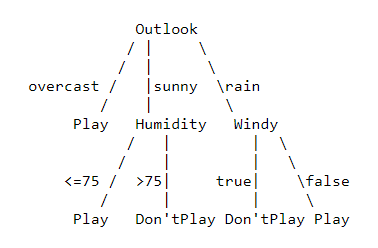# ID3: Iterative Dichotomiser 3, C4.5, CART

There are various types of algorithm available named ID3, C4.5, CART, CHAID, QUEST, GUIDE, CRUISE, and CTREE. Here we are looking to three most commonly used one.

Following up from our previous article on Decision Tree, here we have few commonly used techniques.

Starting from ID3

• Categorical Independent Variables
• Class Labels of Predicted Class is given
• Start with finding root node which is the variable that gives maximum Information Gain. This partitions data into say m parts.
• Next leaving the variable already considered at root node, divide each subtree to arrive at variable giving maximum information gain.

C4.5 Algorithm address few problems in ID3.

• handles missing data
• Unavailable values of attributes :
In building a decision tree we can deal with training sets that have records with unknown attribute values by evaluating the gain, or the gain ratio, for an attribute by considering only the records where that attribute is defined.
In using a decision tree, we can classify records that have unknown attribute values by estimating the probability of the various possible results. Example consider the following tree, where we need to predict for record having ‘outlook’ as ‘sunny’, ‘humidity’ as ‘unknown’.Training Data and Corresponding Decision Tree

To classify our observation, we move through sunny branch of Outlook, then looking at training data we have 2 data points(humidity≤75) and 3 points(Humidity>75). Therefor probability of (play, don’t play) is (2/5, 3/5).

• Continuous attribute value ranges: Say that attribute Ci has a continuous range. We examine the values for this attribute in the training set. Say they are, in increasing order, A1, A2, .., Am. Then for each value Aj, j=1,2,..m, we partition the records into those that have Ci values up to and including Aj, and those that have values greater than Aj. For each of these partitions we compute the gain, or gain ratio, and choose the partition that maximizes the gain. Just like we split above example for Humidity ≥75
• Pruning of decision trees: It is done by replacing a whole subtree by a leaf node. The replacement takes place if a decision rule establishes that the expected error rate in the subtree is greater than in the single leaf. Winston shows how to use Fisher’s exact test to determine if the category attribute is truly dependent on a non-categorical attribute. If it is not, then the non-categorical attribute need not appear in the current path of the decision tree. Quinlan and Breiman suggest more sophisticated pruning heuristics. Read My article here.
• Rule derivation: It is easy to derive a rule set from a decision tree: write a rule for each path in the decision tree from the root to a leaf. In that rule the left-hand side is easily built from the label of the nodes and the labels of the arcs.

CART over others

CART is a further refinement in C4.5 where

• it can deal with continuous dependent and independent variables both.
• uses Multiclass Gini Index Adaptation instead of Entropy or Information Gain. Read my article on Impurity Measure here.
• constructs a sequence of subtrees, and then uses cross validation to estimate misclassification of each subtree. Then it picks the one with least cost.
• can handle outliers

In nutshell, I found a beautiful table.

# My Social Spaces

Problem Solver, Data Science, Actuarial Science, Knowledge Sharer, Hardcore Googler

## More from Shaily jain

Problem Solver, Data Science, Actuarial Science, Knowledge Sharer, Hardcore Googler